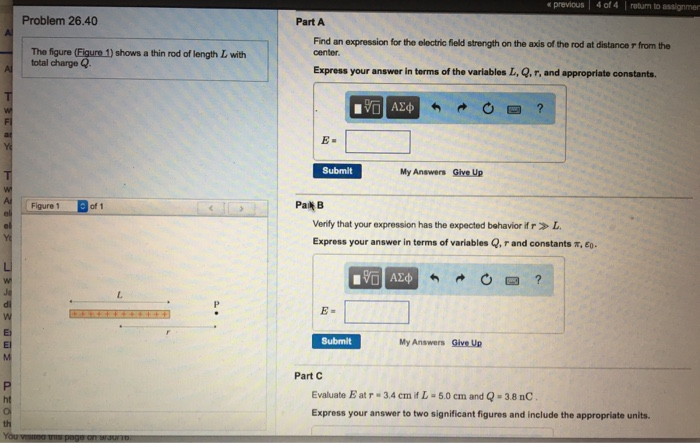# Question Solved1 AnswerThe figure (figure 1) shows a thin rod of length L with total charge Q. Find an expression for the electric field strength on the axis of the rod at distance r from the center. Express your answer in terms of the variables L, Q, r, and appropriate constants. Verify that your expression has the expected behavior if r >> L. Express your answer In terms of variables Q, r and constants pi, epsilon. Evaluate E at r = 3.4 cm if L = 5.0 cm and Q = 3.8 nC. Express your answer to two significant figures and Include the appropriate units.66EOCK The Asker · Other NSMTranscribed Image Text: The figure (figure 1) shows a thin rod of length L with total charge Q. Find an expression for the electric field strength on the axis of the rod at distance r from the center. Express your answer in terms of the variables L, Q, r, and appropriate constants. Verify that your expression has the expected behavior if r >> L. Express your answer In terms of variables Q, r and constants pi, epsilon. Evaluate E at r = 3.4 cm if L = 5.0 cm and Q = 3.8 nC. Express your answer to two significant figures and Include the appropriate units.
More
Transcribed Image Text: The figure (figure 1) shows a thin rod of length L with total charge Q. Find an expression for the electric field strength on the axis of the rod at distance r from the center. Express your answer in terms of the variables L, Q, r, and appropriate constants. Verify that your expression has the expected behavior if r >> L. Express your answer In terms of variables Q, r and constants pi, epsilon. Evaluate E at r = 3.4 cm if L = 5.0 cm and Q = 3.8 nC. Express your answer to two significant figures and Include the appropriate units.